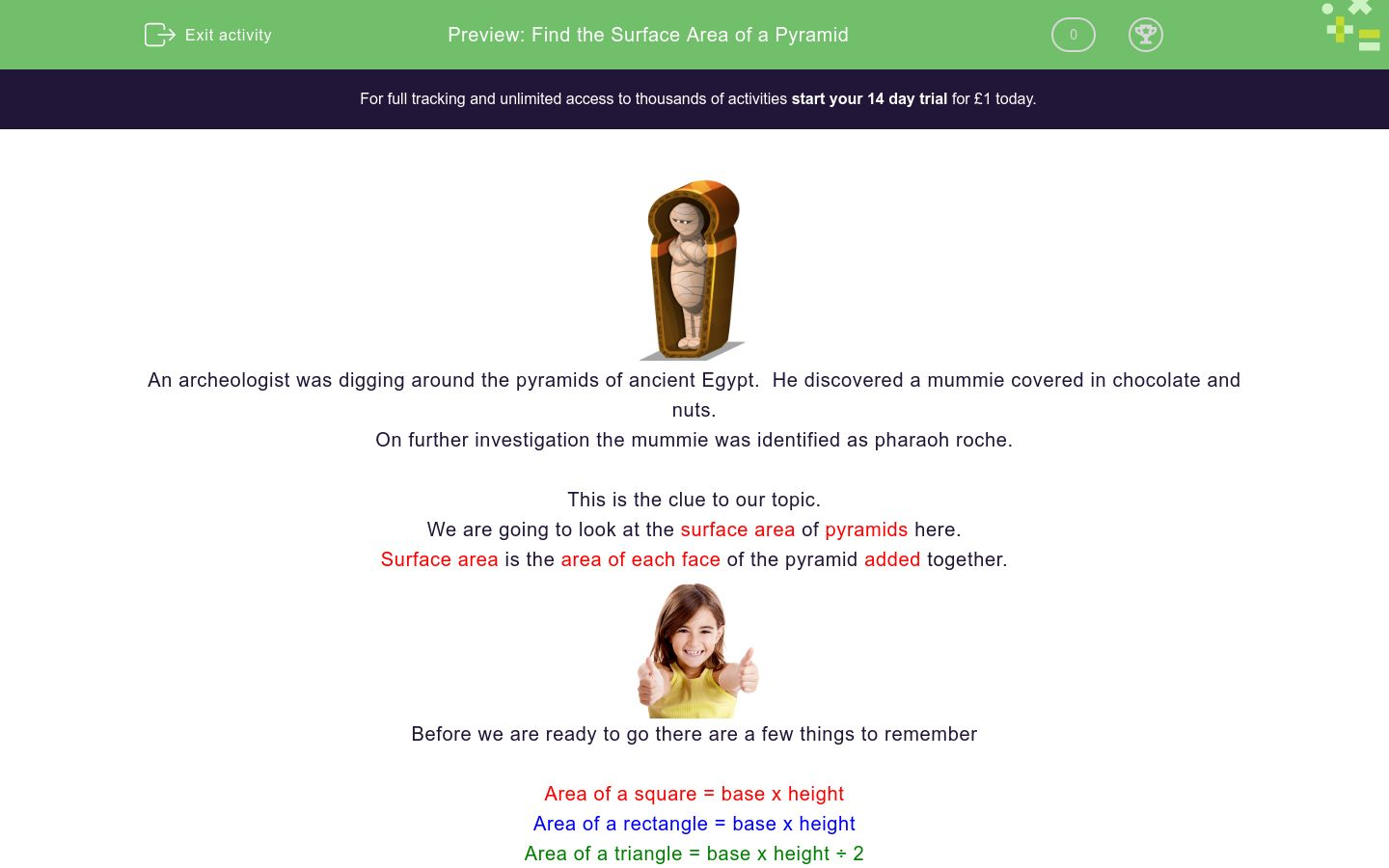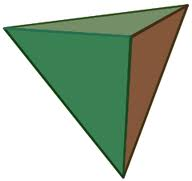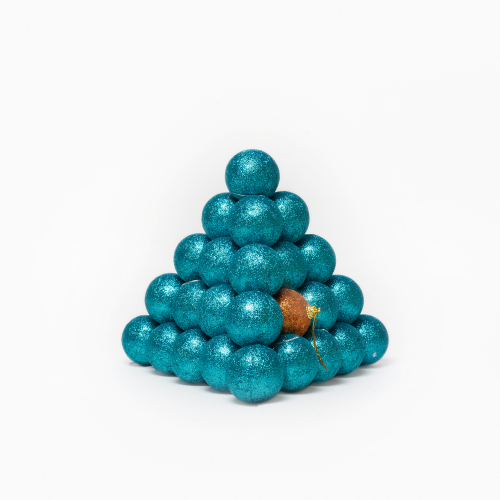# Find the Surface Area of a Pyramid

In this worksheet, students will learn the formula for finding the surface area of a pyramid and be able to apply it.Key stage:  KS 4

GCSE Subjects:   Maths

GCSE Boards:   AQA, Eduqas, Pearson Edexcel, OCR

Curriculum topic:   Geometry and Measures, Mensuration

Curriculum subtopic:   Mensuration and Calculation, Volume and Surface Area Calculations

Difficulty level:### QUESTION 1 of 10An archeologist was digging around the pyramids of ancient Egypt.  He discovered a mummie covered in chocolate and nuts.

On further investigation the mummie was identified as pharaoh roche.

This is the clue to our topic.

We are going to look at the surface area of pyramids here.

Surface area is the area of each face of the pyramid added together.Before we are ready to go there are a few things to remember

Area of a square = base x height

Area of a rectangle = base x height

Area of a triangle = base x height ÷ 2

Example 1This pyramid has a side length of 4 cm and it's height is 5 cm.

To find the surface area of this square based pyramid we

1. Calculate the area of the base  4 x 4 = 16 cm²

2. Find the area of one of the triangular sides  4 x 5 ÷ 2 = 10 cm²

3.  There are four sides to the pyramid so 10 x 4 = 40 cm²

4. Add the total  area of the sides to the area of the base =  16 + 40 = 56 cm²

Example 2This triangular based pyramid has base 6 cm height 8 cm

To find the  surface area of this triangular based pyramid we

1. Calculate the area of the base  6 x 8 ÷ 2 = 24 cm²

2.  There are four sides to the pyramid  (the base and three sides) so 24 x 4 = 96 cm²The sides base has sides of 5 cm and 6 cm. The height of the pyramid 6 cm.

To find the surface area of this rectangular based pyramid we

1. Calculate the area of the base  5 x 6 = 30 cm²

2.  We find the area of the triangle with the base of 5 cm, 5 x 6  ÷ 2 = 15 cm²

3.  The face opposite has the same area so multiply by 2. 15 x 2 = 30 cm²

4.  Now find the area of the triangle with the base of 6 cm. 6 x 6  ÷ 2 = 18 cm²

5. The face opposite has the same area so multiply by 2. 18 x 2 = 36 cm²Where do Pharaoh's like to eat?

Pizza Tut

Hopefully this has made you smile as you start the activities.

Find the surface area of this square based pyramid.Side length 5 cm Height 8 cm.

105 cm²

110 cm²

115 cm²

120 cm²

Find the surface area of this rectangular based pyramid.Side length 3 cm  and 4 cm Height 7 cm.

105 cm²

110 cm²

115 cm²

120 cm²

Find the surface area of this triangular based pyramid.Base length 6 cm and Height 9 cm.

Find the surface area of this square based pyramid.The rectangular based  pyramid has base of 2 cm and 3 cm. Its height is 5 cm.

The square based pyramid has a base of 4 cm and a height of 6 cm

What is the difference in surface area?Find the area of this triangular based pyramid.

Base length 9 cm, height 11 cm

 108 cm² 198 cm² 118 cm² 98 cm² Surface AreaWhat is the total surface area of these pyramids.

The yellow pyramid has base of 7 cm and a height of 8 cm.

The blue pyramid has a base of 7 cm and a height of 7 cm.

 266 cm² 216 cm² 236 cm² 206cm² Total surface areaWhat is the  surface area of this pyramid.

Base of 6 cm and a height of 11 cm.

The surface area of this pyramid is 132 cm² 123 cm²

Find the surface area of this rectangular based pyramid.Side length 6 cm  and 3 cm Height 5 cm.

The surface area of this pyramid is 54cm², 63 cm², 73 cm²Find the area of the square based pyramid, that has side of 22 m and a height of 18 m.

• Question 1

Find the surface area of this square based pyramid.Side length 5 cm Height 8 cm.

105 cm²
EDDIE SAYS
Did you remember to find the area of the base first. 5 x 5 = 25 cm² Next find the area of 1 triangle 5 x 8 ÷ 2 = 20cm² Multiply the area of the triangle by 4. 20 x 4 = 80 cm² Now add this to the area of the base 80 + 25 = 105 cm² By the way, what music do mummies listen to? Rap
• Question 2

Find the surface area of this rectangular based pyramid.Side length 3 cm  and 4 cm Height 7 cm.

105 cm²
EDDIE SAYS
Did you remember to find the area of the base first. 3 x 4 = 12 cm² Next find the area of 1 triangle 3 x 7÷ 2 = 10.5 cm² There are two triangles of this size so 10.5 x 2 = 21 cm² Next find the area of the other triangle 4 x 7÷ 2 = 14 cm² 14 x 2 = 28 cm² Now add everything together 12 + 21 + 28 = 61 cm²
• Question 3

Find the surface area of this triangular based pyramid.Base length 6 cm and Height 9 cm.

108
EDDIE SAYS
Find the area of 1 triangle 6 x 9 ÷ 2 = 27 cm² There are four of them in total = 27 x 4 = 108 cm² Brilliant stuff. It makes life so much easier when there is only one area to work out doesn't it.
• Question 4

Find the surface area of this square based pyramid.75
EDDIE SAYS
What no units? Actually there are. Count the baubles. Find the area of the base first. 5 x 5 = 25 units² Next find the area of 1 triangle 5 x 5 ÷ 2 = 12.5 units² There are four triangles of this size so 12.5 x 4 = 50 units² Now add to the base area Now add everything together 50 + 25 =75 units²
• Question 5The rectangular based  pyramid has base of 2 cm and 3 cm. Its height is 5 cm.

The square based pyramid has a base of 4 cm and a height of 6 cm

What is the difference in surface area?

EDDIE SAYS
Rectangular based pyramid. 2 x 3 = 6 cm² One triangle 3 x 5 ÷ 2 x 2 = 15 cm² Next find the area of the other triangle 2 x 5 ÷ 2 x 10 cm² Now add together 6 + 15 + 10 = 31cm² Square based pyramid base 4 x 4 = 16 cm² area of one triangle 4 x 6 ÷ 2 = 12 cm² 12 x 4= 48 cm² Now add everything together 48 + 16 = 64 cm² The word difference suggests subtraction so 64 - 31 = 33 cm²
• Question 6Find the area of this triangular based pyramid.

Base length 9 cm, height 11 cm

 108 cm² 198 cm² 118 cm² 98 cm² Surface Area
EDDIE SAYS
Oh yes I like these because there is not so much to do. Hooray. Find the area of one triangle 9 x 11 ÷ 2 = 49.5 cm² Now just multiply your answer by 4 49.5 x 4 = 198 cm² Bingo
• Question 7What is the total surface area of these pyramids.

The yellow pyramid has base of 7 cm and a height of 8 cm.

The blue pyramid has a base of 7 cm and a height of 7 cm.

 266 cm² 216 cm² 236 cm² 206cm² Total surface area
EDDIE SAYS
Here you are not told the shape so you have to be extra vigilant. Surface area of the yellow square based pyramid 7 x 8 = 56 cm² base Triangle 7 x 8 ÷ 2 x 4 = 112 cm² 112 + 56 = 168 cm² Surface area of the blue triangular based pyramid 7 x 7 ÷ 2 x 4 = 98 cm² Total surface area means add them together 168 + 98 = 266 cm² What did the Pharaoh say when he saw the pyramid? Mummies home.
• Question 8What is the  surface area of this pyramid.

Base of 6 cm and a height of 11 cm.

The surface area of this pyramid is 132 cm² 123 cm²
EDDIE SAYS
Are you getting the point of this yet.... Please don't groan, they can get worse 6 x 11 ÷ 2 x 4 = 132 cm²
• Question 9

Find the surface area of this rectangular based pyramid.Side length 6 cm  and 3 cm Height 5 cm.

The surface area of this pyramid is 54cm², 63 cm², 73 cm²
EDDIE SAYS
Area of the base is 6 x 3 = 18 cm² Area of triangle 1 is 3 x 5 ÷2 = 7.5 x 2 = 15 cm² Area of triangle 12 is 6 x 5 ÷2 = 15 x 2 = 30 cm² Now add up 18 + 15 + 30 = 63 cm² Hopefully you can see the point of all this practice.
• Question 10Find the area of the square based pyramid, that has side of 22 m and a height of 18 m.

990
EDDIE SAYS
Don't you think the sphinx guarding that pyramid has big feet. Hopefully they won't get in the way of us finding the surface area. Find the base first 22 x 22 = 484 cm² Now find the area of one triangle 22 x 18 ÷ 2 = 198 cm² 198 x 4 = 792 cm² 792 + 198 = 990 cm²
---- OR ----

Sign up for a £1 trial so you can track and measure your child's progress on this activity.

### What is EdPlace?

We're your National Curriculum aligned online education content provider helping each child succeed in English, maths and science from year 1 to GCSE. With an EdPlace account you’ll be able to track and measure progress, helping each child achieve their best. We build confidence and attainment by personalising each child’s learning at a level that suits them.

Get started